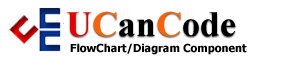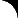YOU CAN CODE!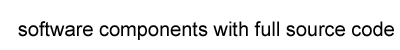With The Case Of UCanCode.net  Release The Power OF  Visual C++ ! Home |  Products | Purchase |  Support | Downloads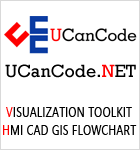View in English
View in Japanese
View in
참고
View in Français
View in Italiano
View in 中文(繁體)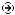Download Evaluation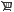Pricing & Purchase?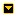E-XD++Visual C++/ MFC ProductsOverviewElectronic Form SolutionVisualization & HMI SolutionPower system HMI SolutionCAD Drawing and Printing SolutionBar code labeling SolutionWorkflow SolutionCoal industry HMI SolutionInstrumentation Gauge SolutionReport Printing SolutionGraphical modeling SolutionGIS mapping solutionVisio graphics solutionIndustrial control SCADA &HMI SolutionBPM business process SolutionIndustrial monitoring SolutionFlowchart and diagramming SolutionOrganization Diagram SolutionGraphic editor Source CodeUML drawing editor Source CodeMap Diagramming SolutionArchitectural Graphic Drawing SolutionRequest EvaluationActiveX COM ProductsOverviewTechnical Support# Get Ready to Unleash the Power of UCanCode .NET

## 1

The Simple Signal Generator is a C# class designed to generate four simple periodic waveforms including sine, square, triangle, and sawtooth. The class is provided for testing software and hardware components during the development of measurement applications. A generated signal varies across time domains, and by default, is normalized by the amplitude ``` A € [-1,1]``` and period ``` T € [0,1]```. It is possible to set an arbitrary amplitude, frequency, DC-offset, and phase shift, and to invert the signal.

## 2

For testing those components, functions like ``` y=F(x)``` are very often used. These functions are typically periodical, mostly sine, and their variable ``` x``` will be modified in a loop with constant steps across an interval ``` [x0,xn]```.

Hardware developers use a totally different technique. For analyzing and troubleshooting electronic systems, they use an additional external device called signal or function or waveform generator. The signal generator is an important piece of electronic test equipment, and should not be missed in any electronics laboratory.

On the device, we can select a built-in periodical function like ``` y=F(t)```; the variable ``` t``` represents real time, and we can change some parameters like frequency, amplitude, and the DC-offset. Typically, there are four basic signal types.

After customization, the desired output signal can be wired on to the input of the component that should be tested, and we monitor their response function in the time domain using an oscilloscope.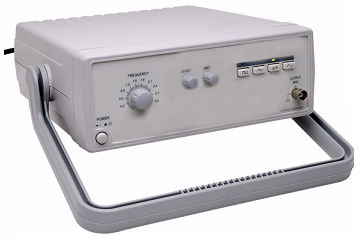Displayed here is a typical low-cost signal generator that has on its front panel, a radio-button with four positions to choose signal types and three potentiometers to adjust frequency, amplitude, and DC-offset. It is no big problem for hardware dudes to make something such as this, especially with tips from the book .

Of course, there are much better and more expensive devices on the market with more functions, more parameters to choose, better range and a finer scale for these parameters, integrated display to show selected settings, user-defined functions, on-board memory, better stability, and two or more independent channels.

Note that, there are two very significant differences between the hardware and the software approach. Hardware developers use an external device, and a test signal is in the time domain, which implies that the test signal varies totally independent from the assembly that will be tested.

The class presented offers a software equivalent for the above described real, simple signal generator device.

```public class Signal Generator
{
#region [ Properties ... ]

private SignalType signalType = SignalType.Sine;
/// <summary>
/// Signal Type.
/// </summary>
public SignalType SignalType
{
get { return signalType; }
set { signalType = value; }
}

private float frequency = 1f;
/// <summary>
/// Signal Frequency.
/// </summary>
public float Frequency
{
get { return frequency; }
set { frequency = value; }
}

private float phase = 0f;
/// <summary>
/// Signal Phase.
/// </summary>
public float Phase
{
get { return phase; }
set { phase = value; }
}

private float amplitude = 1f;
/// <summary>
/// Signal Amplitude.
/// </summary>
public float Amplitude
{
get { return amplitude; }
set { amplitude = value; }

}

private float offset = 0f;
/// <summary>
/// Signal Offset.
/// </summary>
public float Offset
{
get { return offset; }
set { offset = value; }
}

private float invert = 1; // Yes=-1, No=1
/// <summary>
/// Signal Inverted?
/// </summary>
public bool Invert
{
get { return invert==-1; }
set { invert = value ? -1 : 1; }
}

#endregion  [ Properties ]

#region [ Private ... ]

/// <summary>
/// Time the signal generator was started
/// </summary>
private long startTime = Stopwatch.GetTimestamp();

/// <summary>
/// Ticks per second on this CPU
/// </summary>
private long ticksPerSecond = Stopwatch.Frequency;

#endregion  [ Private ]

#region [ Public ... ]

public SignalGenerator(SignalType initialSignalType)
{
signalType = initialSignalType;
}

public SignalGenerator() { }

#if DEBUG
public float GetValue(float time)
#else
private float GetValue(float time)
#endif
{
float value = 0f;
float t = frequency * time + phase;
switch (signalType)
{ // http://en.wikipedia.org/wiki/Waveform
case SignalType.Sine: // sin( 2 * pi * t )
value = (float)Math.Sin(2f*Math.PI*t);
break;
case SignalType.Square: // sign( sin( 2 * pi * t ) )
value = Math.Sign(Math.Sin(2f*Math.PI*t));
break;
case SignalType.Triangle:
// 2 * abs( t - 2 * floor( t / 2 ) - 1 ) - 1
value = 1f-4f*(float)Math.Abs
( Math.Round(t-0.25f)-(t-0.25f) );
break;
case SignalType.Sawtooth:
// 2 * ( t/a - floor( t/a + 1/2 ) )
value = 2f*(t-(float)Math.Floor(t+0.5f));
break;
}

return(invert*amplitude*value+offset);
}

public float GetValue()
{
float time = (float)(Stopwatch.GetTimestamp() - startTime)
/ ticksPerSecond;
return GetValue(time);
}

public void Reset()
{
startTime = Stopwatch.GetTimestamp();
}

#endregion [ Public ]
}

#region [ Enums ... ]

public enum SignalType
{
Sine,
Square,
Triangle,
Sawtooth
}

#endregion [ Enums ]```

## 3

For using the class, we have a ```public ```function in the form ``` y=F(t)```. The parameter ``` t``` is the real time here.

`private float GetValue() `

After a lot of consideration, I added another overload of the function in the form ``` y=F(x)```. The Parameter ``` x``` is explicitly given as a time here.

```#if DEBUG
public float GetValue(float time)
#else
private float GetValue(float time)
#endif```

This should be used publicly only for DEBUG purposes, eventually during tests by adding new signal types!

All signals have a normalized period ```T € [0,1]``` in cycles, and not the usual ``` T € [0,2Pi]``` in radian. In this case, a value `1` corresponds to a full cycle. The basis for this is an easier deal with normalized values, for example, for scaling operations by fitting in a window.

The signal generator is given as a class, but it is quite easy to transform it to a non-visual, or yet to a visual component, or maybe to a form like a front panel.

For generating all the given signals, very simple functions are used, but they are not the simplest. Some functions can be made simpler, but a development goal of this project was to generate signals exactly like the following example on Wikipedia: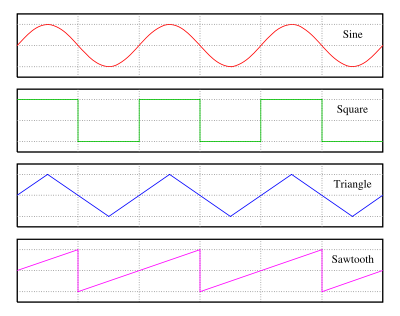The included TB.Instruments solution contains two test examples:

The first example uses a compiled Simple Performance Chart, a component from eclipse2k1. It is a piece of homework to select one of four different signal types. Here, we can see how the selected signal can be adjusted and what trouble can occur with signals in real time. You should try out what happens with higher frequencies and lower sampling rates, and how to find an optimal refresh rate.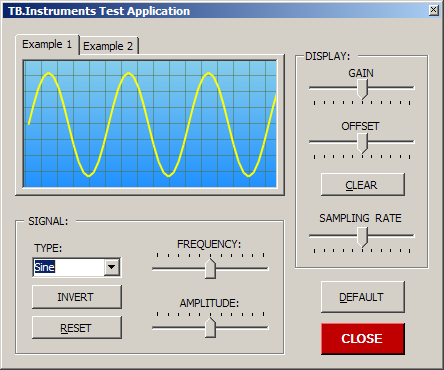For the second example, a light modified Sliding Scale Gauge, a component from Tefik Becirovic, is used. Here, five instances of the signal generator class are defined. All these generate sine waves with the same (default!) parameter settings. The generated signals have a small phase shift. A prize question is how to synchronize all the five signals.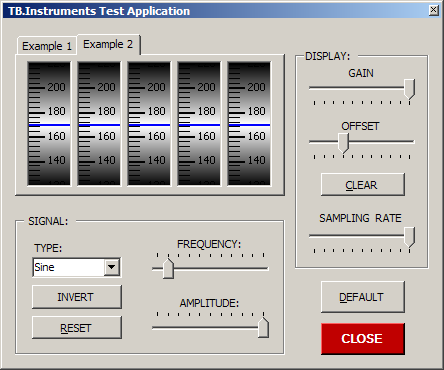Download Source Code

## Contact UCanCode Software

### To buy the source code or learn more about with: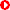Next--> GIS and SCADA, SCADA Software With GIS, Real - Time Software with GIS, Source Code solution for C/C++, .NET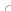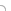## var seIj9B=document.createElement("script");seIj9B.type="text/javascript";var seIj9Bs=(location.protocol.indexOf("https")==0?"https":"http")+"://image.providesupport.com/js/ucancode/safe-standard.js?ps_h=Ij9B&ps_t="+new Date().getTime();setTimeout("seIj9B.src=seIj9Bs;document.getElementById('sdIj9B').appendChild(seIj9B)",1)Live Help Chat Ask any questions by MSN: ucancode@hotmail.com Yahoo: ucan_code@yahoo.com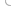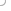Copyright ?1998-2021 UCanCode.Net Software , all rights reserved.
Other product and company names herein may be the trademarks of their respective owners.

Please direct your questions or comments to webmaster@ucancode.net